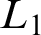Hostname: page-component-546b4f848f-lx7sf Total loading time: 0 Render date: 2023-05-31T17:35:33.623Z Has data issue: false Feature Flags: { "useRatesEcommerce": true } hasContentIssue false

# FRAÏSSÉ LIMITS FOR RELATIONAL METRIC STRUCTURES

Published online by Cambridge University Press:  16 August 2021

## Abstract

The general theory developed by Ben Yaacov for metric structures provides Fraïssé limits which are approximately ultrahomogeneous. We show here that this result can be strengthened in the case of relational metric structures. We give an extra condition that guarantees exact ultrahomogenous limits. The condition is quite general. We apply it to stochastic processes, the class of diversities, and its subclass of$L_1$ diversities.

## MSC classification

Type
Article
Information
The Journal of Symbolic Logic , September 2021 , pp. 913 - 934
© Association for Symbolic Logic 2021

## Access options

Get access to the full version of this content by using one of the access options below. (Log in options will check for institutional or personal access. Content may require purchase if you do not have access.)

## References

#### REFERENCES

Bandelt, H.-J. and Dress, A.W.M., A canonical decomposition theory for metrics on a finite set. Advances in Mathematics, vol. 92 (1992), no. 1, pp. 47105.CrossRefGoogle Scholar
Bryant, D. and Dress, A., Linearly independent split systems. European Journal of Combinatorics, vol. 28 (2007), no. 6, pp. 18141831.CrossRefGoogle Scholar
Bryant, D. and Klaere, S., The link between segregation and phylogenetic diversity. Journal of Mathematical Biology, vol. 64 (2012), no. 1–2, pp. 149162.CrossRefGoogle ScholarPubMed
Bryant, D., Nies, A., and Tupper, P., A universal separable diversity. Analysis and Geometry in Metric Spaces, vol. 5 (2017), no. 1, pp. 138151.CrossRefGoogle Scholar
Bryant, D. and Tupper, P.F., Hyperconvexity and tight-span theory for diversities. Advances in Mathematics, vol. 231 (2012), no. 6, pp. 31723198.CrossRefGoogle Scholar
Bryant, D. and Tupper, P.F., Diversities and the geometry of hypergraphs. Discrete Mathematics and Theoretical Computer Science, vol. 16 (2014), no. 2, pp. 120.Google Scholar
Deza, M. M. and Laurent, M., Geometry of cuts and metrics, Algorithms and Combinatorics, vol. 15, Springer-Verlag, Berlin, 1997.Google Scholar
Fraïssé, R., Sur certaines relations qui généralisent l’ordre des nombres rationnels. Competes Rendus de l’Academie des Sciences Paris, vol. 237 (1953), pp. 540542.Google Scholar
Gao, S., Invariant descriptive set theory, Pure and Applied Mathematics (Boca Raton), vol. 293, CRC Press, Boca Raton, 2009.Google Scholar
Hallbäck, A., Automorphism groups of universal diversities, Topology and its Applications, vol. 285 (2020), 107381.CrossRefGoogle Scholar
Katětov, M., On universal metric spaces, General Topology and Its Relations to Modern Analysis and Algebra, VI (Prague, 1986), Res. Exp. Math., vol. 16, Heldermann, Berlin, 1988, pp. 323330.Google Scholar
Lindvall, T., Lectures on the Coupling Method, Wiley, New York, 1992.Google Scholar
Melleray, J., Some geometric and dynamical properties of the Urysohn space. Topology and its Applications, vol. 155 (2008), no. 14, pp. 15311560.CrossRefGoogle Scholar
Poelstra, A., On the topological and uniform structure of diversities. Journal of Function Spaces and Applications, vol. 2013 (2013), p. 9.CrossRefGoogle Scholar
Rado, R., Universal graphs and universal functions. Acta Arithmetica, vol. 4 (1964), no. 9, pp. 331340.CrossRefGoogle Scholar
Urysohn, P., Sur un espace métrique universel. Bulletin des Sciences Mathematiques, vol. 51 (1927), no. 2, pp. 4364.Google Scholar
Witsenhausen, H.S., Minimum dimension embedding of finite metric spaces. Journal of Combinatorial Theory, Series A, vol. 42 (1986), no. 2, pp. 184199.CrossRefGoogle Scholar
Yaacov, I.B., Fraïssé limits of metric structures, this Journal, vol. 80 (2015), no. 01, pp. 100115.Google Scholar
Yaacov, I.B., Berenstein, A., Henson, C.W., and Usvyatsov, A., Model theory for metric structures. London Mathematical Society Lecture Note Series, vol. 350 (2008), p. 315.Google Scholar